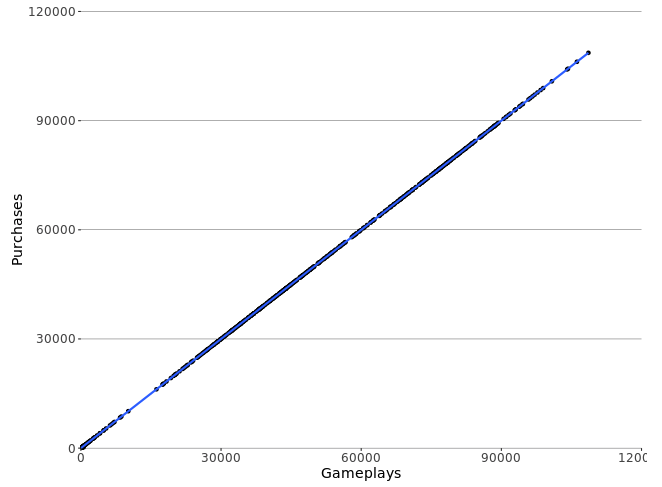Using ggplot2 for charts with a similar look to Periscope Data's default charting

If you have a mix of visualizations using Periscope Data's default charting options and R/Python charts, ensuring a uniform look gives a cohesive flow to the entire dashboard.

By playing around with ggplot2's customization options, we can get closer to the Periscope charting look:

output <- output +
scale_x_continuous(expand = c(0, 0)) +
scale_y_continuous(expand = c(0, 0))

output <- output +
theme(panel.background = element_rect(fill = "transparent"),
panel.grid.major.x = element_blank(),
panel.grid.major.y =element_line(size=.15, color="gray15"),
axis.text=element_text(family='NimbusSan',size=12),
axis.title.x=element_text(family='NimbusSan',size=14),
axis.title.y=element_text(family='NimbusSan',size=14))

Here's the result:Any other customizations you would add on here? Comment below!

(Python equivalent to come)